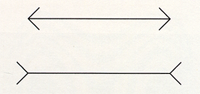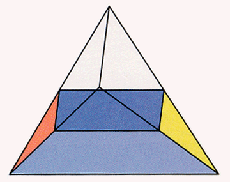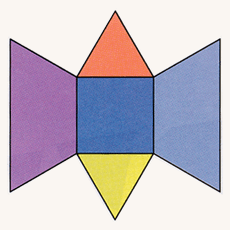## Slicing the Triangular Pyramid

We can use these same slicing techniques to investigate figures besides cubes. Consider the slices of a triangular pyramid, or tetrahedron. Slicing parallel to one of the triangular faces, we start with a triangle determined by three of the four points, and these slices become smaller triangles shrinking down to the fourth vertex.Arrows at the ends of equal-length segments produce the illusion that one is longer.

If we slice by planes parallel to one of the edges, we get rectangles, and in the central position, a square. This slicing sequence leads to an interesting two-piece puzzle. The slice containing the square separates the tetrahedron into two parts of exactly the same shape. You can make a paper model of the two pieces of this decomposition by folding up the pattern at the side of this page. Many people find it difficult to put these two identical pieces together to form a triangular pyramid. Even when they place the two square faces together, most often they hold the pieces so that the longest edges are parallel instead of perpendicular as they should be. The difficulty seems to be related to the three-dimensional equivalent of the optical illusion that makes two lines of equal length seem different if we put arrows on the ends. The presence of the longest sides makes the square faces appear to be rectangles with unequal sides.Slicing the tetrahedron edge first produces a square slice in the middle (left); fold-out pattern for one-half of the subdivided tetrahedron (right).